#### Theory of TTL Logic Gate Series

Circuit Structures:

In general, the functions of different stages of any gate are identical to those of inverter and NAND gates. It is good to recall, though, that many logic functions are implemented by the real connections made in a circuit structure. Recall, for illustration, the input phase of the inverter.

The input transistor T1 acts as a current steering and amplifying phase. It is T2 which essentially functions the inversion. Therefore, in TTL structures, the configuration of transistors shown in figure below will always execute an inverting operation.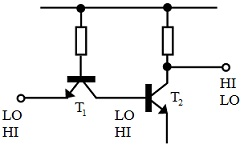Figure: Inverting Structure

Remember, as well, the input structure of NAND gate. The input combination of multiple emitters functions a logical AND operation on inputs in the current steering mechanism. This is T2 which performs the inversion to provide an overall NAND operation.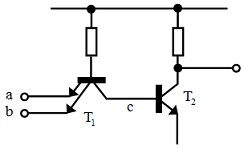Figure: ‘AND’ Structure

Let consider a final structure where two transistors are basically joined in parallel with a common load as shown in figure below. Principally, if either transistor is turned on, it will conduct and draw current via the load making the output go low. Only whenever both transistors are OFF, tending to make the output HI individually, will the output really be HI. This is an AND operation and the connection is termed to as a wired and connection. This circuit structure is equal to two inverters on the input of an AND gate that is logically equivalent to an NOR structure as can be seen from the figure and table shown below: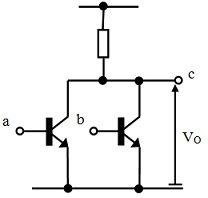Figure: ‘OR’ Structure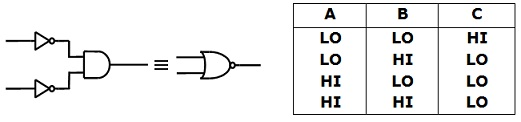Figure: Logical Equivalent of ‘Or’ ing (NOR) Structure

Standard TTL 74-Series Logic Gates:

The figures below show complete circuit schematic diagrams for some of the fundamental logic gates in the Standard TTL 7400 series with usual component values comprised. The diodes shown on the inputs are protection diodes for input transistors. Such prevent negative voltages being developed at input terminals due to ringing on inductive lines employed to join gates altogether. Large, negative spikes at input would tend to over bias the base-emitter junction of input transistors and destroy them. Such voltages are clamped to a diode drop by input protection diodes.

A) Inverter 7404

B) NAND Gate 7400

C) NOR Gate 7402

Transistor pairs T1 + T2 and T5 + T6 form the inverters to inputs. The parallel connection of the collectors of T2 and T6 gives a wired AND function as previously explained. The output phase, T3 + T4, is as before. This gives the function:

a‾.b‾ = (a+b)‾

That is the NOR operation.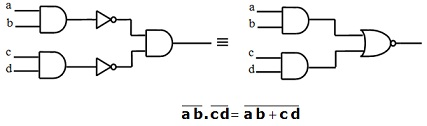D) AND-OR-INVERT Gate 7451:

This is really a combination of NAND and NOR gate structures. The multiple emitter input transistors T1 and T5 execute an AND function on their individual inputs. The following transistors T2 and T6 invert the AND functions. The parallel connection of T2 and T6 gives the wired AND function as in NOR gate. The overall condition is equivalent to that shown in figure below. The notable feature of this gate is that it permits Boolean functions to be implemented in sum-of-products form in a single gate with a propagation delay equivalent to that of an inverter. The inversion of function can be corrected for by implementing 0 terms of function instead of 1s.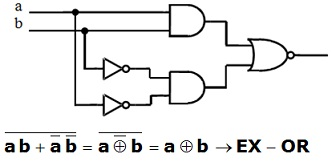Figure: Logical Equivalent of AND-OR-INVERT Structure

E) EXCLUSIVE-OR Gate 7486:

This can be seen that T1 to T6 of this structure is similar to that of A-O-I Gate and executes the same logical operation. Transistors T7 + T8 and T9 + T10 just comprise an inversion on one pair of inputs. The circuit is logically equal to the structure shown in figure below that is seen to offer an exclusive-OR operation.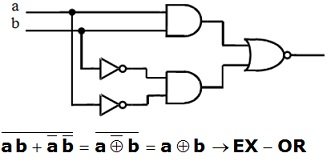Figure: Logical Equivalent of Exclusive OR Gate Structure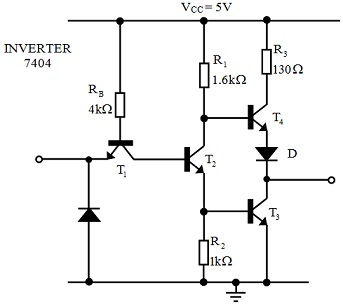Figure: Schematic Diagram of 7404 Standard TTL Inverter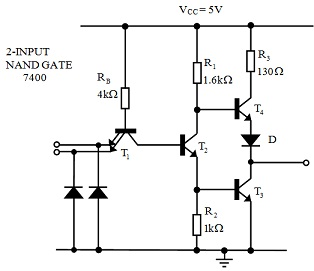Figure: Schematic Diagram of 7400 Standard TTL NAND Gate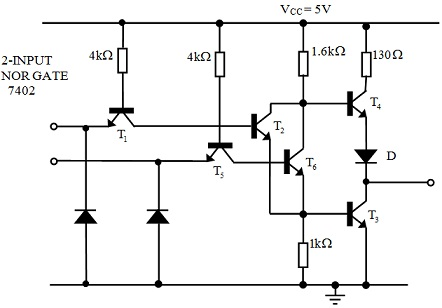Figure: Schematic Diagram of 7402 Standard TTL NOR Gate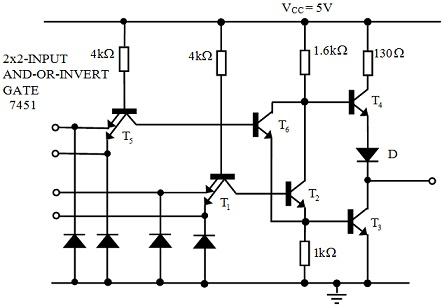Figure: Schematic Diagram of 7451 Standard TTL AND-OR-INVERT Gate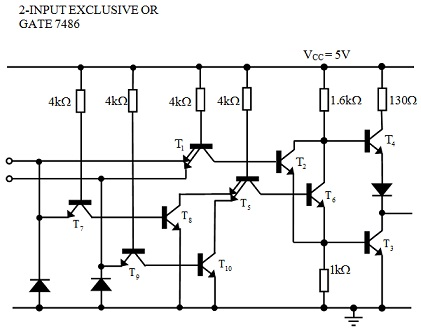Figure: Schematic Diagram of 7486 Standard TTL EXCLUSIVE-OR Gate

Latest technology based Electrical Engineering Online Tutoring Assistance

Tutors, at the www.tutorsglobe.com, take pledge to provide full satisfaction and assurance in Electrical Engineering help via online tutoring. Students are getting 100% satisfaction by online tutors across the globe. Here you can get homework help for Electrical Engineering, project ideas and tutorials. We provide email based Electrical Engineering help. You can join us to ask queries 24x7 with live, experienced and qualified online tutors specialized in Electrical Engineering. Through Online Tutoring, you would be able to complete your homework or assignments at your home. Tutors at the TutorsGlobe are committed to provide the best quality online tutoring assistance for Electrical Engineering Homework help and assignment help services. They use their experience, as they have solved thousands of the Electrical Engineering assignments, which may help you to solve your complex issues of Electrical Engineering. TutorsGlobe assure for the best quality compliance to your homework. Compromise with quality is not in our dictionary. If we feel that we are not able to provide the homework help as per the deadline or given instruction by the student, we refund the money of the student without any delay.

2015 ©TutorsGlobe All rights reserved. TutorsGlobe Rated 4.8/5 based on 34139 reviews.